+

# Valuing Multiple Cash Flows

Author: Sophia Tutorial
##### Description:

Understand how to calculate the future and present value of multiple cash flows.

(more)### Developing Effective Teams

*No strings attached. This college course is 100% free and is worth 1 semester credit.

37 Sophia partners guarantee credit transfer.

299 Institutions have accepted or given pre-approval for credit transfer.

* The American Council on Education's College Credit Recommendation Service (ACE Credit®) has evaluated and recommended college credit for 32 of Sophia’s online courses. Many different colleges and universities consider ACE CREDIT recommendations in determining the applicability to their course and degree programs.

Tutorial

what's covered
In this lesson, you will learn how to calculate the future and present value of multiple cash flows. Specifically, this lesson will cover:
1. Future Value, Multiple Flows
2. Present Value, Multiple Flows
3. Calculating Present Value from Future Value

## 1. Future Value, Multiple Flows

Finding the future value (FV) of multiple cash flows means that there are more than one payment/investment, and a business wants to find the total FV at a certain point in time. These payments can have varying sizes, occur at varying times, and earn varying interest rates, but they all have a certain value at a specific time in the future.

The first step in finding the FV of multiple cash flows is to define when the future is. Once that is done, you can determine the FV of each cash flow using the formula below. Then, simply add all of the future values together.

formula

FV of Multiple Cash Flows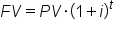Manually calculating the FV of each cash flow and then summing them together can be a tedious process. If the cash flows are irregular, don’t happen at regular intervals, or earn different interest rates, there isn’t a special way to find the total FV.

However, if the cash flows do happen at regular intervals, are a fixed size, and earn a uniform interest rate, there is an easier way to find the total FV. Investments that have these three traits are called annuities.

There are formulas to find the FV of an annuity depending on some characteristics, such as whether the payments occur at the beginning or end of each period. There is another module that goes through exactly how to calculate the FV of annuities.

If the multiple cash flows are a part of an annuity, you’re in luck; there is a simple way to find the FV. If the cash flows aren’t uniform, don’t occur at fixed intervals, or earn different interest rates, the only way to find the FV is to find the FV of each cash flow and then add them together.

## 2. Present Value, Multiple Flows

The PV of multiple cash flows follows the same logic as the FV of multiple cash flows. The PV of multiple cash flows is simply the sum of the present values of each individual cash flow. We can use the following formula to find the PV:

formula

PV of Multiple Cash Flows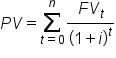Each cash flow must be discounted to the same point in time.

EXAMPLE

You cannot sum the PV of two loans at the beginning of the loans if one starts in 2012 and one starts in 2014. If you want to find the PV in 2012, you need to discount the second loan an additional two years, even though it doesn’t start until 2014.

The calculations get markedly simpler if the cash flows make up an annuity. In order to be an annuity, the cash flows need to have three traits:

• Constant payment size
• Payments occur at fixed intervals
• A constant interest rate
Things may get slightly messy if there are multiple annuities, and you need to discount them to a date before the beginning of the payments.

### IN CONTEXT

Suppose there are two sets of cash flows which you determine are both annuities. The first extends from 1/1/14 to 1/1/16, and the second extends from 1/1/15 to 1/1/17. You want to find the total PV of all the cash flows on 1/1/13.

The annuity formulas are good for determining the PV at the date of the inception of the annuity. That means that it’s not enough to simply plug in the payment size, interest rate, and number of periods between 1/1/13 and the end of the annuities. If you do, that supposes that both annuities begin on 1/1/13, but neither do. Instead, you have to first find the PV of the first annuity on 1/1/14 and the second on 1/1/15 because that’s when the annuities begin.

You now have two present values, but both are still in the future. You then can discount those present values as if they were single sums, to 1/1/13.

Unfortunately, if the cash flows do not fit the characteristics of an annuity, there isn’t a simple way to find the PV of multiple cash flows; each cash flow must be discounted and then all of the PVs must be summed together.
term to know

Discounting
The process of finding the present value using the discount rate.

## 3. Calculating Present Value from Future Value

A corporation must decide whether to introduce a new product line. The new product will have start-up expenditures, operational expenditures, and then it will have associated incoming cash receipts (sales) and disbursements (cash paid for materials, supplies, direct labor, maintenance, repairs, and direct overhead) over 12 years.

This project will have an immediate (t=0) cash outflow of \$100,000, which might include all cash paid for the machinery, transportation-in and set-up expenditures, and initial employee training disbursements.

The annual net cash flow (receipts less disbursements) from this new line for years 1-12 is forecast as follows:

Year 1: -\$54,672
Year 2: -\$39,161
Year 3: \$3,054
Year 4: \$7,128
Year 5: \$25,927
Year 6: \$28,838
Year 7: \$46,088
Year 8: \$77,076
Year 9: \$46,726
Year 10: \$76,852
Year 11: \$132,332
Year 12: \$166,047
This is reflecting two years of running deficits as experience and sales are built up, with net cash receipts forecast positive after that.

At the end of the 12 years, it’s estimated that the entire line becomes obsolete and its scrap value just covers all the removal and disposal expenditures. All values are after-tax, and the required rate of return is given to be 10%. (This also makes the simplifying assumption that the net cash received or paid is lumped into a single transaction occurring on the last day of each year.)

The present value (PV) can be calculated for each year:

Time PV
T=0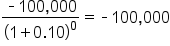T=1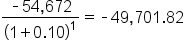T=2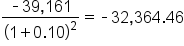T=3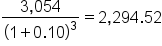T=4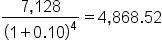T=5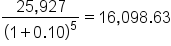T=6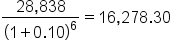T=7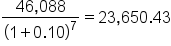T=8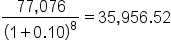T=9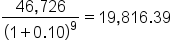T=10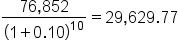T=11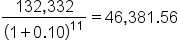T=12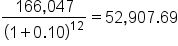SUM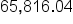The sum of all these present values is the net present value, which equals \$65,816.04. Since the NPV is greater than zero, it would be better to invest in the project than to do nothing, and the corporation should invest in this project if there is no alternative with a higher NPV.

term to know

Net Present Value
The present value of a project or an investment decision determined by summing the discounted incoming and outgoing future cash flows resulting from the decision.
summary
In this lesson, you learned that you can calculate the future value of multiple flows by applying the formula for future value to each investment and adding the results together. Similarly, you can find the present value of multiple flows by summing up the present value of each cash flow. Calculating present value from future value allows companies to make decisions about long-term investments, such as new product lines.

Best of luck in your learning!

Source: THIS CONTENT HAS BEEN ADAPTED FROM LUMEN LEARNING'S “Valuing Multiple Cash Flows” TUTORIAL.

Terms to Know
Discounting

The process of finding the present value using the discount rate.

Net Present Value

The present value of a project or an investment decision determined by summing the discounted incoming and outgoing future cash flows resulting from the decision.

Formulas to Know
FV of Multiple Cash FlowsPV of Multiple Cash FlowsRating